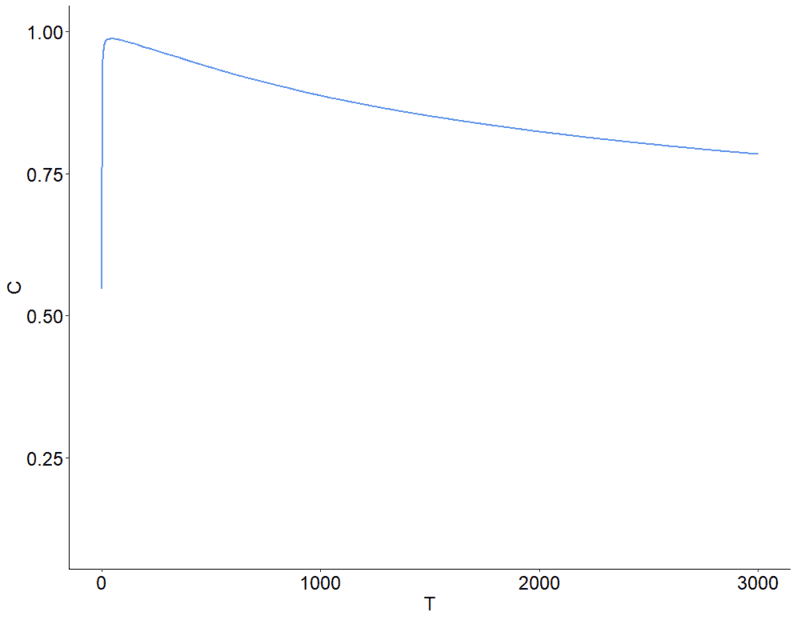# I want to smooth this function plot

• B
Hi,
I have the following function, which is computed by: (x+n)/(x+y+n+m),
where x, y are real numbers
n, m are natural numbersWhat techniques I can use to smooth the function preventing it to jump up or down at an early stage.

Thanks

Office_Shredder
Staff Emeritus
Gold Member
I'm confused, your axis on the bottom is labeled as T, what does that have to do with your function?

I kind of assumed x and y were both inputs into your function, so I'm confused how you graphed it like this in general.

•I'm confused, your axis on the bottom is labeled as T, what does that have to do with your function?

I kind of assumed x and y were both inputs into your function, so I'm confused how you graphed it like this in general.
Thank you. T represents the time. It seems that n increases quickly in the early timesteps. I am still thinking of ways to use T in the formula.

jbriggs444
Homework Helper
Thank you. T represents the time. It seems that n increases quickly in the early timesteps. I am still thinking of ways to use T in the formula.
You have still not told us what it is that you have graphed.

•Mark44
Mentor
The graph in post #1 makes no sense given your formula. The labels on the graph are C and T, but the formula appears to be a function of x and y, as well as m and n.

Disregarding the m and n terms for the moment, if you have ##f(x, y) = \frac x {x + y}##, the graph will be a surface in three dimensions, with a discontinuity along the line y = -x.

Last edited:
•fresh_42
Mentor
The formula, as well as the graph, are already smooth within their domain.

•Thank you. Yes, I just want to slow down the jump at the beginning.

berkeman
Mentor
Thank you. Yes, I just want to slow down the jump at the beginning.

x(T)
n(T)
y(T)
m(T)

•fresh_42

x(T)
n(T)
y(T)
m(T)
x,n,y,m are variables that are changing over time

berkeman
Mentor
x,n,y,m are variables
You have a plot with them! Show us the funtion that you used to generate the plot please.

You have a plot with them! Show us the funtion that you used to generate the plot please.
I construct the function but not fully sure if it is the best way to put all the variables together. I am building a simulation and trying to model human decisions but my model is very simple. The function is above with the question. x and y represent positive and negative personal experiences. n and m represent positive and negative opinions on social media. I assume the combination of all variables specifies the probability of consumption. Because at the beginning of the simulation, social media has 0 opinions, at step 1, multiple opinions are posted.

fresh_42
Mentor
You have plotted a function ##F\, : \, \mathbb{R}^+\longrightarrow [0,1]## which means for any value ##T\in \mathbb{R}^+## you plotted a point ##(T,F(T)).## Our question is: What is ##F##? It depends only on ##T##, so how do we get from ##\dfrac{x+n}{x+n+y+m}## to ##F(T)##?

You have plotted a function ##F\, : \, \mathbb{R}^+\longrightarrow [0,1]## which means for any value ##T\in \mathbb{R}^+## you plotted a point ##(T,F(T)).## Our question is: What is ##F##? It depends only on ##T##, so how do we get from ##\dfrac{x+n}{x+n+y+m}## to ##F(T)##?
F depends on all x,y,n,m, and T also.

berkeman
Mentor
Sigh. Your obfuscations will get you nowhere...

Whatever. If you don't like the first point, then just don't plot it. How's that for a solution?

••